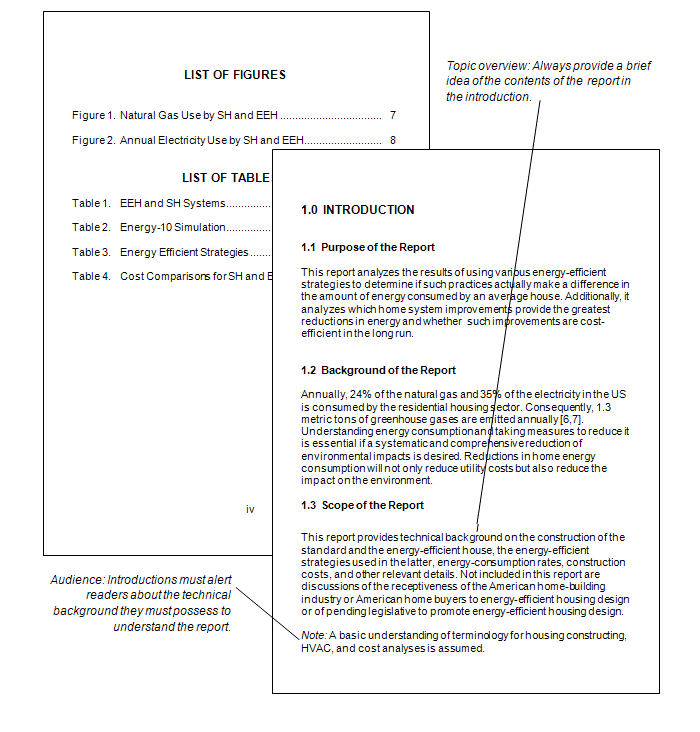# 10.5: List of figures and tables

$$\newcommand{\vecs}{\overset { \rightharpoonup} {\mathbf{#1}} }$$ $$\newcommand{\vecd}{\overset{-\!-\!\rightharpoonup}{\vphantom{a}\smash {#1}}}$$$$\newcommand{\id}{\mathrm{id}}$$ $$\newcommand{\Span}{\mathrm{span}}$$ $$\newcommand{\kernel}{\mathrm{null}\,}$$ $$\newcommand{\range}{\mathrm{range}\,}$$ $$\newcommand{\RealPart}{\mathrm{Re}}$$ $$\newcommand{\ImaginaryPart}{\mathrm{Im}}$$ $$\newcommand{\Argument}{\mathrm{Arg}}$$ $$\newcommand{\norm}{\| #1 \|}$$ $$\newcommand{\inner}{\langle #1, #2 \rangle}$$ $$\newcommand{\Span}{\mathrm{span}}$$ $$\newcommand{\id}{\mathrm{id}}$$ $$\newcommand{\Span}{\mathrm{span}}$$ $$\newcommand{\kernel}{\mathrm{null}\,}$$ $$\newcommand{\range}{\mathrm{range}\,}$$ $$\newcommand{\RealPart}{\mathrm{Re}}$$ $$\newcommand{\ImaginaryPart}{\mathrm{Im}}$$ $$\newcommand{\Argument}{\mathrm{Arg}}$$ $$\newcommand{\norm}{\| #1 \|}$$ $$\newcommand{\inner}{\langle #1, #2 \rangle}$$ $$\newcommand{\Span}{\mathrm{span}}$$$$\newcommand{\AA}{\unicode[.8,0]{x212B}}$$

If your document has more than two figures or tables create a separate list of figures. The list of figures has many of the same design considerations as the table of contents. Readers use the list of figures to quickly find the illustrations, diagrams, tables, and charts in your report.

Complications arise when you have both tables and figures. Strictly speaking, figures are illustrations, drawings, photographs, graphs, and charts. Tables are rows and columns of words and numbers; they are not considered figures.

For longer reports that contain dozens of figures and tables each, create separate lists of figures and tables. Put them together on the same page if they fit, as shown in the illustration below. You can combine the two lists under the heading, “List of Figures and Tables,” and identify the items as figure or table as is done in the illustration below.List of figures and tables followed by the introduction. If there are no tables, make it “List of Figures.” In a technical writing course, ask your instructor if the decimal-numbering style for headings is required.